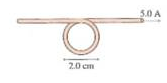# Problem: What are the strength and direction of the magnetic field at the center of the loop?

###### FREE Expert Solution

Magnetic field due to a loop:

$\overline{){\mathbf{B}}{\mathbf{=}}\frac{{\mathbf{\mu }}_{\mathbf{0}}\mathbf{I}}{\mathbf{2}\mathbf{r}}}$

Magnetic field due to a straight conductor:

$\overline{){\mathbf{B}}{\mathbf{=}}\frac{{\mathbf{\mu }}_{\mathbf{0}}\mathbf{I}}{\mathbf{2}\mathbit{\pi }\mathbf{r}}}$

The magnetic field at the center of the loop is the sum of the field due to the loop and a straight conductor.###### Problem Details

What are the strength and direction of the magnetic field at the center of the loop?